## LetsPlayMaths.Com

WELCOME TO THE WORLD OF MATHEMATICS

# Class 4 Length

Measurement of Length

Conversion of Bigger Units into Smaller Units

Conversion of Smaller Units into Bigger Units

Subtraction of Length

Multiplication of Length

Division of Length

Length Test

Length Worksheet

## Measurement of Length

In class III, we have learnt about kilometer (km), meter (m) and centimeter (cm). Here we will learn about decimeter and millimeter.

1 kilometer = 1000 meter

1 meter = 100 centimeter

If we divide 1 meter into 100 equal parts, then each part is called centimeter.

If we divide 1 meter into 10 equal parts, then each part is called decimeter.

1 meter = 10 decimeter

If we divide 1 centimeter into 10 equal parts, then each part is called millimeter.

1 centimeter = 10 millimeter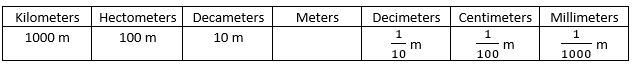## Conversion of Bigger Units into Smaller Units

Let’s look at some examples given below.

Example 1. Convert 9 kilometers into meters.

Solution. We know 1 km = 1000 m
9 km = 9 X 1000 m = 9000 m
So, 9 km is equal to 9000 meters.

Example 2. Convert 7 km 250 m into meters.

Solution. 7 km 250 m = 7 km + 250 m = 7 × 1000 m + 250 m = 7000 m + 250 m = 7250 m
So, 7 km 250 m is equal to 7250 m

Example 3. Convert 5 m 9 cm into centimeters.

Solution. 5 m 9 cm = 5 × 100 cm + 9 cm = 500 cm + 9 cm = 509 cm
So, 5 m 9 cm is equal to 509 cm.

Example 4. Convert 120 dm into centimeters.

Solution. We know 1 dm = 10 cm
120 dm = 120 × 10 cm = 1200 cm
So, 120 dm is equal to 1200 cm.

Example 5. Convert 5 dm 7 cm into centimeters.

Solution. 5 dm 7 cm = 5 dm + 7 cm = 5 × 10 cm + 7 cm = 50 cm + 7 cm = 57 cm
So, 5dm 7 cm is equal to 57 cm.

Example 6. Convert 8 m 5 dm into centimeter.

Solution. 8 m 5 dm = 8 × 100 cm + 5 × 10 cm = 800 cm + 50 cm = 850 cm
So, 8 m 5 dm is equal to 850 cm.

## Conversion of Smaller Units into Bigger Units

Divide the number of millimeter by 10 to Convert millimeter into centimeter. Let’s have a look at some example.

Example 1. Convert 65 mm into cm.

Solution. 65mm ÷ 10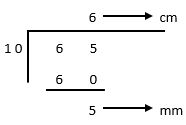So, the answer is 6 cm 5 mm, or we can write as 6.5 cm.

Divide the number of centimeter by 10 to convert centimeter into decimeter. Let's have a look at some example.

Example 1. Convert 274 cm into dm.

Solution. 274 cm ÷ 10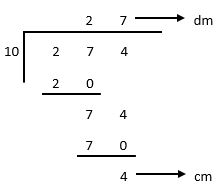So, the answer is 27 dm 4 cm or 27.4 dm

Divide the number of millimeter by 100 to convert millimeter into decimeter.

Example 1. Convert 345 mm into dm.

Solution. 345 mm ÷ 100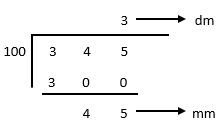So, the answer is 3 dm 45 mm or 3.45 dm.

Divide the number of centimeter by 100 to convert centimeter into meter.

Example 1. Convert 607 cm into meters.

Solution. 607 mm ÷ 100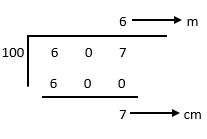So, the answer is 6 m 7 cm or 6.07 m.

Divide the number of meters by 1000 to convert meter into kilometers.

Example 1. Convert 3875 meters into kilometers.

Solution. 3875 ÷ 1000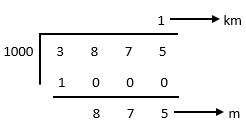So, the answer is 1 km 875 m or 1.875 km.

## Addition of Length

We have learnt the addition of length measures in class III. Here, we will use the same methods to add the bigger quantities. We can add meters, decimeters, centimeters and millimeters like normal numbers. Let’s have a look at some examples.

Example 1. Add 64 cm 8 mm and 23 cm 5 mm.

Solution. Arrange both the numbers in tabular format as shown below.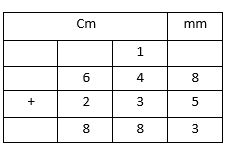So, the answer is 88 cm 3 mm.

Example 2. Add 245 km 56 m and 158 km 7 m.

Solution. Arrange both the numbers in tabular format as shown below. Here meters should be written in 3 digit format,
that is 56 m should be written as 056 m.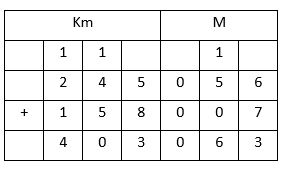So, the answer is 403 km 63 m.

Example 3. Add 24 m 3 dm 6 cm and 7 m 4 dm 2 cm.

Solution. Arrange both the numbers in tabular format as shown below.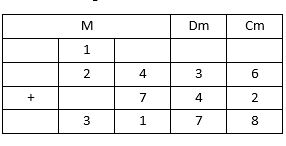So, the answer is 31 m 7 dm 8 cm.

## Subtraction of Length

We have learnt subtraction of length measures in class III. Here, we will use the same methods to subtract the bigger quantities. We can subtract meters, decimeters, centimeters and millimeters like normal numbers. Let’s have a look at some examples.

Example 1. Subtract 56 cm 9 mm from 90 cm.

Solution. Arrange both the numbers in tabular format as shown below.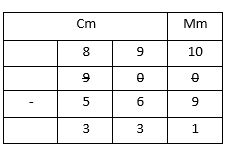So, the answer is 33 cm 1 mm.

Example 2. Subtract 245 km 473 m from 654 km 100 m.

Solution. Arrange both the numbers in tabular format as shown below. Here meters should be written in 3 digit format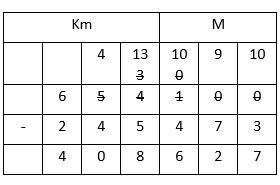So, the answer is 408 km 627 m.

Example 3. Subtract 25 m 4 dm 7 cm from 45 m 9 cm.

Solution. Arrange both the numbers in tabular format as shown below.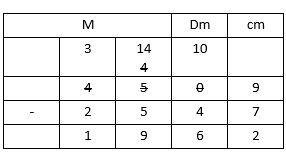So, the answer is 19 m 6 dm 2 cm.

## Multiplication of Length

We can multiply kilometers, meters, decimeters and centimeters as normal multiplication. Let’s have a look at some examples.

Example 1. Multiply 5 m 15 cm by 3 cm.

Solution. Arrange both the numbers in tabular format as shown below.So, the answer is 15 m 45 cm.

Example 2. Multiply 6 m 4 dm 5 cm by 4.

Solution. Arrange both the numbers in tabular format as shown below.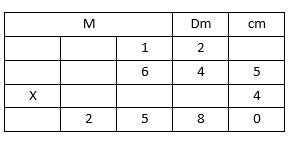So, the answer is 25 m 8 dm.

Example 3. Multiply 21 km 562 m by 5.

Solution. Arrange both the numbers in tabular format as shown below. Here meters should be written in 3 digit format.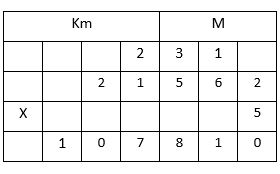So, the answer is 107 km 810 m.

## Division of Length

We can divide kilometers, meters, decimeters and centimeters as normal multiplication. Let’s have a look at some examples.

Example 1. Divide 460 m 45 cm by 5.

Solution.So, the answer is 92 m 9 cm.

Example 2. Divide 35 km 310 m by 3.

Solution.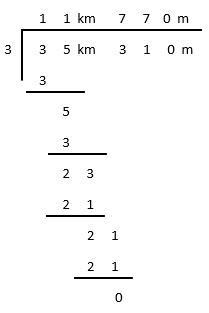So, the answer is 11 km 770 m.

Length Test - 1

Length Test - 2

## Class-4 Length Worksheet

Length Worksheet - 1

Length Worksheet - 2

Length Worksheet - 3

Length Worksheet - 4

Length Worksheet - 5